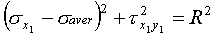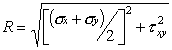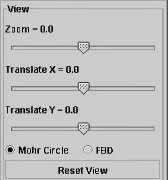search UMD   This siteView vectors in 3D. If the applet doesnt load, you may need to install the appropriate plugins, a link to the plugins can be foundwhereandwhich is the equation of a circle   σ x 1 τ x 1 y 1   as the coordinates.  The circle has radius R, and its center has coordinates as   σ x 1 = σ aver     and     τ x 1 y 1 = 0.Various inputs can be given using sliders on this panel. The negative values shows the compression of the corresponding plane. Outputs values can be viewed in the panel and the corresponding points on the Mohr Circle are Color-Coded.View can be set from this panel. Zoom, translate and highlighting the Mohr Circle/ Free Body Diagram can be controlled through this panel.This is a sample screen in which Mohr Circle is highlighted.This is a sample screen in which Free Body Diagram is highlighted. We can see the negative value (Compression) of Normal Stress in y-direction is represented with arrows pointing inside in the Free Body Diagram.